## Anderson acceleration, Sinkhorn and Multi-Marginal Euler¶

### Author: F.-X. Vialard.¶

I thank Antoine Levitt for sharing his idea of applying AA to Sinkhorn algorithm. I was curious to see how it performs on some multimarginal problems.

### Anderson acceleration¶

Anderson acceleration (AA) is a method to accelerate fixed point iterations $x_{k+1} = G(x_{k+1})$ of a function $G: \mathbb{R}^d \mapsto \mathbb{R}^d$. This method has been introduced by Anderson in 1965 and has been applied recently to problems in electronic structure computations, and it is also called DIIS in computational chemistry. Some theoretical results are available in, among others, Anderson acceleration for fixed point iterations by Walker and Ni and also Convergence analysis for Anderson acceleration by Toth and Kelley. Recently, AA has been applied in Anderson acceleration of the alternating projections method for computing the nearest correlation matrix by Higham and Strabic.

Sinkhorn algorithm and entropic multi-marginal optimal transport is an application of the alternating projection method Iterative Bregman Projections for Regularized Transportation Problems by Benamou et al.

Let's apply AA to entropic multimarginal optimal transport.

In :
### Anderson acceleration scheme as written in
### Anderson acceleration for fixed point iterations by Walker and Ni.

function AndersonAcceleration2(FixedPointFunc::Function,x::Array{Float64,1},iterations::Int64,Lnumber::Int64)
### NOT OPTIMIZED
nx = size(x)
### Allocate variables
IteratesMatrixTemp = zeros(nx,Lnumber+1)
DeltaIteratesMatrix = zeros(nx,Lnumber)

ResidualsMatrixTemp = zeros(nx,Lnumber+1)
DeltaResidualsMatrix = zeros(nx,Lnumber)

### Build Matrices Data on the first Lnumber steps
for i=1:Lnumber+1
ResidualsMatrixTemp[:,i] = -deepcopy(x)
x = FixedPointFunc(x)
ResidualsMatrixTemp[:,i] += x
IteratesMatrixTemp[:,i] = deepcopy(x)
end
old_iterate = IteratesMatrixTemp[:,Lnumber+1]
old_residual = ResidualsMatrixTemp[:,Lnumber+1]
for i=1:Lnumber
DeltaResidualsMatrix[:,i] = ResidualsMatrixTemp[:,i+1] - ResidualsMatrixTemp[:,i]
DeltaIteratesMatrix[:,i] = IteratesMatrixTemp[:,i+1] - IteratesMatrixTemp[:,i]
end
### Iterations of Anderson
for i in 1:iterations
### compute weights and update current iterate
weights = DeltaResidualsMatrix\old_residual
x = old_iterate - DeltaIteratesMatrix*weights
new_iterate = FixedPointFunc(x)
### update matrices
index = (i%Lnumber)+1
DeltaIteratesMatrix[:,index] = new_iterate-old_iterate
DeltaResidualsMatrix[:,index] = new_iterate-x - old_residual
### update residuals
old_residual = new_iterate - x
old_iterate = new_iterate
end
return x
end

Out:
AndersonAcceleration2 (generic function with 1 method)

### Simple Sinkhorn fixed point iteration for entropic optimal transport¶

Given a matrix $K$ (possibly rectangular) with positive entries and positive vectors $\mu$ and $\nu$ (possibly of different sizes, representing probability densities) which have the same total sum (1 for a probability density), Sinkhorn algorithm outputs two diagonal matrices $D_1$ and $D_2$ such that $D_1 K D_2$ has sums on lines and columns equal respectively to $\mu$ and $\nu$ (see the corresponding numerical tour of Gabriel Peyré).

In :
### Crude implementation of Sinkhorn on the multiplicative weights in the matrix P.
abstract Parameters

type ParamsForFixedPoint <: Parameters
nu::Array{Float64,1} ### marginal 1
mu::Array{Float64,1} ### marginal 2
coupling::Array{Float64,2} ### kernel matrix
convergence::Array{Float64,1}
end
function FixedPoint(P::Array{Float64,2},p::Parameters)
P[:,1] = (p.nu)./((p.coupling)*P[:,2])
push!(p.convergence,log(norm(p.mu - P[:,2].*(p.coupling'*P[:,1]))))
P[:,2] = (p.mu)./((p.coupling)'*P[:,1])
return P
end

### Sinkhorn is actually naturally formulated on the log variables. Therefore, it is important
### to have access to it for acceleration
### We just use the previous function and apply the log and exp.
function FixedPointLog(P::Array{Float64,2},p::Parameters)
P = exp(P)
P = FixedPoint(P,p)
P = log(P)
end

Out:
FixedPointLog (generic function with 1 method)

### Implementation of 3 simple strategies¶

We will use three different optimization schemes:

• the first one is the simple fixed point iteration method which uses as the fixed point function the alternate projection (Sinkhorn) implemented above (also in the multi-marginal case below).
• The second use AA after some iterations iterations_burn with the simple fixed point iteration, then use only AA.
• The last alternates between the two. The idea is to save computations by avoiding using AA too often and hoping that the convergence rate is still good.
In :
### Optimization routines
function OptimizationSimpleFixedPoint(FixedPointFunc::Function,P_init,iterations,p::Parameters)
P = deepcopy(P_init)
for k=1:iterations
P = FixedPointFunc(P,p)
end
return P,p.convergence
end

function OptimizationAnderson(FixedPointFunc::Function,P_init,iterations_burn,iterations_anderson,memoryNumber,p::Parameters)
P = deepcopy(P_init)
function functiontemp(x)
y = reshape(x,size(P_init))
z = FixedPointFunc(y,p)
return reshape(z,length(P_init))
end
for k=1:iterations_burn
P = FixedPointFunc(P,p)
end
P = AndersonAcceleration2(functiontemp,reshape(P,length(P)),iterations_anderson,memoryNumber)
return reshape(P,size(P_init)),p.convergence
end

function OptimizationAndersonMixed(FixedPointFunc::Function,P_init,iterations_simple,iterations_anderson,number_of_steps,memoryNumber,p::Parameters)
P = deepcopy(P_init)
function functiontemp(x)
y = reshape(x,size(P))
z = FixedPointFunc(y,p)
return reshape(z,length(P))
end
for r in 1:number_of_steps
for t in 1:iterations_simple
P = FixedPointFunc(P,p)
end

P = AndersonAcceleration2(functiontemp,reshape(P,length(P_init)),iterations_anderson,memoryNumber)
P = reshape(P,size(P_init))
end
return reshape(P,size(P_init)),p.convergence
end

Out:
OptimizationAndersonMixed (generic function with 1 method)
In :
### Helper to generate some gaussian"like" data ###
function GenerateGaussians(mean, std,x)
function Gaussians(y)
exp(-((y-mean)/std)^2)
end
w = broadcast(Gaussians,x) + 0.12 * ones(length(x))
w/sum(w)
end

function GenerateCoupling(x,sigma)
n = length(x)
coupling = zeros(n,n)
cost = zeros(n,n)
for j=1:n
for i=1:n
cost[i,j] = (x[i]-x[j])^2
coupling[i,j] = exp(-(cost[i,j])/sigma^2)
end
end
return coupling
end

Out:
GenerateCoupling (generic function with 1 method)

### Standard sinkhorn convergence for different regularization parameters¶

We first use the simple fixed point scheme to show the convergence of Sinkhorn which deteriorates rapidly when the regularization parameter $\sigma$ in the kernel gets smaller, from blue to red. Here, the convergence is an $L^2$ distance checking one of the two marginal constraints.

In :
### Generate some data once for all !
n = 100;
x = linspace(0,1,n);
nu = GenerateGaussians(0.2,0.1^2,x);
mu = GenerateGaussians(0.8,0.1^2,x);
P_init_simple = ones(n,2);
P_init_log = zeros(n,2);

In :
using PyPlot
iterations = 500
results = []
for sigma in [0.5,0.1,0.01]
convergence = []
coupling = GenerateCoupling(x,sigma)
p = ParamsForFixedPoint(nu,mu,coupling,convergence)
P,convergence_simple = OptimizationSimpleFixedPoint(FixedPoint,P_init_simple,iterations,p)
push!(results,convergence_simple)
end
for convergence_result in results
plot(1:length(convergence_result),convergence_result)
end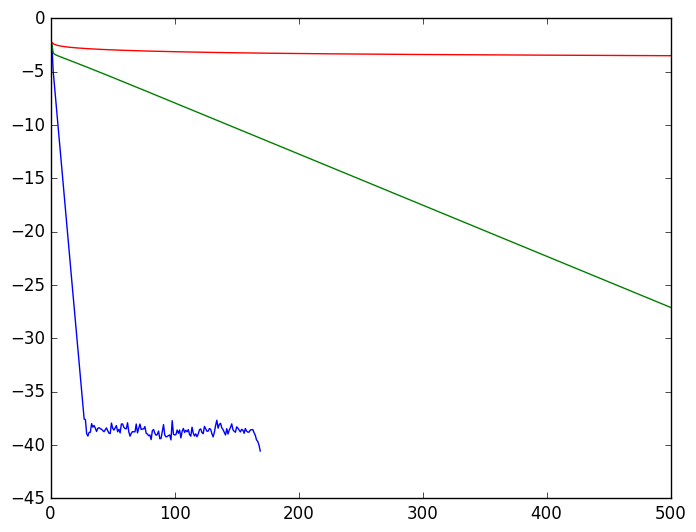### Different memory numbers¶

We now apply AA to a medium range regularization parameter $\sigma$. We apply AA with two different parameter for the memory number. To make the plot clearer, we start AA at two different iterations steps although this impacts also a bit the results.

In :
sigma = 0.03
convergence = []
coupling = GenerateCoupling(x,sigma)
iterations_burn, iterations_anderson = 30, 400
iterations_total = iterations_burn + iterations_anderson
### Change the number of initial iterations
iterations_burn2=50
iterations_anderson2 = iterations_total - iterations_burn2
iterations_burn3=60
iterations_anderson3 = iterations_total - iterations_burn3
convergence_anderson, convergence_simple, convergence_anderson2,convergence_anderson3 = [],[],[],[]
p_anderson, p_simple = ParamsForFixedPoint(nu,mu,coupling,convergence_anderson), ParamsForFixedPoint(nu,mu,coupling,convergence_simple)
p_anderson2 = ParamsForFixedPoint(nu,mu,coupling,convergence_anderson2)
p_anderson3 = ParamsForFixedPoint(nu,mu,coupling,convergence_anderson3)
### Memory number for anderson acceleration:
memoryNumber1 = 20
memoryNumber2 = 10
memoryNumber3 = 5
### Run the optimization
P,convergence_anderson1 = OptimizationAnderson(FixedPointLog,P_init_log,iterations_burn,iterations_anderson,memoryNumber1,p_anderson)
P,convergence_anderson2 = OptimizationAnderson(FixedPointLog,P_init_log,iterations_burn2,iterations_anderson2,memoryNumber2,p_anderson2)
P,convergence_anderson3 = OptimizationAnderson(FixedPointLog,P_init_log,iterations_burn3,iterations_anderson3,memoryNumber3,p_anderson3)

P,convergence_simple = OptimizationSimpleFixedPoint(FixedPoint,P_init_simple,iterations_total,p_simple)

### Plot
plot(1:length(convergence_anderson1),convergence_anderson1)
plot(1:length(convergence_anderson2),convergence_anderson2)
plot(1:length(convergence_anderson3),convergence_anderson3)
plot(1:length(convergence_simple),convergence_simple)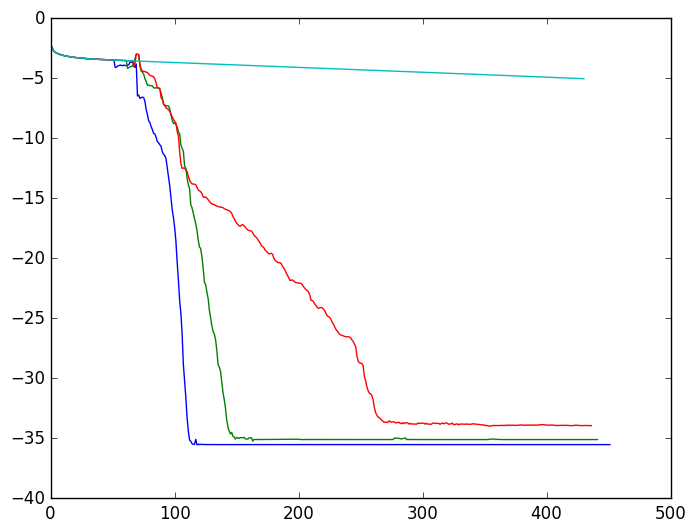Out:
1-element Array{Any,1}:
PyObject <matplotlib.lines.Line2D object at 0x32abfb9d0>

### Smaller regularization parameters¶

Clearly, the results are very positive and the number of memory number can be chosen around 10 in this example, which confirms related results in the literature. Now, we want to go to smaller regularization parameter. For that, we need to implement Sinkhorn in the log domain.

In :
### Implement crude Sinkhorn in log domain
type ParamsForFixedPointLogTrue <: Parameters
nu::Array{Float64,1} ### marginal 1
mu::Array{Float64,1} ### marginal 2
cost::Array{Float64,2} ### kernel matrix
sigmaSquared::Float64
convergence::Array{Float64,1}
end
function FixedPointLogTrue(P::Array{Float64,2},p::ParamsForFixedPointLogTrue)
px = P[:,1]
py = P[:,2]
n = length(p.nu)
m = length(p.mu)
for i=1:n
temp = 0
for j=1:m
temp+=exp(-p.cost[i,j]/p.sigmaSquared  + py[j] + px[i])
end
px[i] += -log(temp) + log(p.nu[i])
end
temp2 = 0
for j=1:m
temp = 0
for i=1:n
temp+=exp(-p.cost[i,j]/p.sigmaSquared + py[j] + px[i])
end
temp2 += abs(temp - p.mu[j])^2
py[j] += - log(temp) + log(p.mu[j])
end
push!(p.convergence,log(sqrt(temp2)))
result = zeros(n,2)

result[:,1] = px
result[:,2] = py
return result
end
function GenerateCost(x,sigma)
coupling = zeros(n,n)
cost = zeros(n,n)
for j=1:n
for i=1:n
cost[i,j] = (x[i]-x[j])^2
coupling[i,j] = exp(-(cost[i,j])/sigma^2)
end
end
return cost
end

WARNING: Method definition (::Type{Main.ParamsForFixedPointLogTrue})(Array{Float64, 1}, Array{Float64, 1}, Array{Float64, 2}, Float64, Array{Float64, 1}) in module Main at In:3 overwritten at In:3.
WARNING: Method definition (::Type{Main.ParamsForFixedPointLogTrue})(Any, Any, Any, Any, Any) in module Main at In:3 overwritten at In:3.
WARNING: Method definition FixedPointLogTrue(Array{Float64, 2}, Main.ParamsForFixedPointLogTrue) in module Main at In:10 overwritten at In:10.
WARNING: Method definition GenerateCost(Any, Any) in module Main at In:38 overwritten at In:38.

Out:
GenerateCost (generic function with 1 method)

### Some instable behaviour of Anderson acceleration¶

We show an example where Anderson acceleration does not really succeed well. This is probably surprising since Sinkhorn algorithm is linearly converging because it is a contraction with respect to a Hilbert metric. However, the minimum has not been

In :
### Generate some data
sigma = 0.005
convergence = []
coupling = GenerateCoupling(x,sigma)
P_init_log, P_init_simple = zeros(n,2), ones(n,2)
iterations_burn, iterations_anderson = 1000, 4000
iterations_total = iterations_burn + iterations_anderson
### Change the number of initial iterations
iterations_burn2=1000
iterations_anderson2 = iterations_total - iterations_burn2
convergence_anderson, convergence_simple, convergence_logtrue, convergence_logtruesimple = [],[],[],[]
p_anderson, p_simple = ParamsForFixedPoint(nu,mu,coupling,convergence_anderson), ParamsForFixedPoint(nu,mu,coupling,convergence_simple)
cost  = GenerateCost(x,sigma)
sigmaSquared = sigma^2
p_logtrue = ParamsForFixedPointLogTrue(nu,mu,cost,sigmaSquared,convergence_logtrue)
p_logtruesimple = ParamsForFixedPointLogTrue(nu,mu,cost,sigmaSquared,convergence_logtruesimple)
### Memory number for anderson acceleration:
memoryNumber = 8

### Run the optimization
P,convergence_logtrue = OptimizationAnderson(FixedPointLogTrue,P_init_log,iterations_burn2,iterations_anderson2,memoryNumber,p_logtrue)
#P,convergence_logfake = OptimizationAnderson(FixedPointLog,P_init_log,iterations_burn2,iterations_anderson2,memoryNumber,p_anderson)
P,convergence_simple = OptimizationSimpleFixedPoint(FixedPointLogTrue,P_init_log,iterations_total,p_logtruesimple)
P,convergence_simplefake = OptimizationSimpleFixedPoint(FixedPointLog,P_init_log,iterations_total,p_simple)

### Plot
plot(1:length(convergence_logtrue),convergence_logtrue)
plot(1:length(convergence_simple),convergence_simple)
#plot(1:length(convergence_logfake),convergence_logfake,"o")
plot(1:length(convergence_simplefake),convergence_simplefake,"o")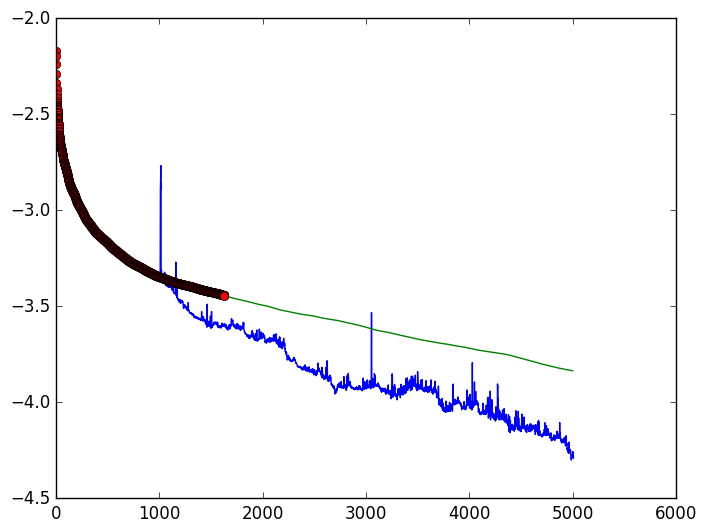Out:
1-element Array{Any,1}:
PyObject <matplotlib.lines.Line2D object at 0x32bf614d0>

### A simple workaround¶

We see that the naive Sinkhorn implementation is not robust with small $\sigma$ since the dotted curve is a collection of NaN after a certain number of iterations. The log-domain implementation makes it more robust.

However, AA does not seem to work as before. It seems that there is a lot of "noise" in the iterations. Let's try to make it more robust and use OptimizationAndersonMixed by alternating between standard fixed point and AA, which will a priori also deteriorate the convergence.

In :
sigma = 0.005
sigmaSquared = sigma^2

coupling = GenerateCoupling(x,sigma)
cost  = GenerateCost(x,sigma)
P_init_log, P_init_simple = zeros(n,2), ones(n,2)
### Memory number for anderson acceleration:
memoryNumber = 8
### Iterations
iterations_anderson, iterations_simple, number_of_steps = 2, 100, 35
iterations_total = (iterations_simple+ iterations_anderson + memoryNumber)*number_of_steps
### Change the number of initial iterations

convergence_anderson_mixed, convergence_simple,convergence_anderson = [],[],[]
p_anderson = ParamsForFixedPointLogTrue(nu,mu,cost,sigmaSquared,convergence_anderson)
p_anderson_mixed = ParamsForFixedPointLogTrue(nu,mu,cost,sigmaSquared,convergence_anderson_mixed)
p_logtruesimple = ParamsForFixedPointLogTrue(nu,mu,cost,sigmaSquared,convergence_simple)

P,convergence_anderson = OptimizationAnderson(FixedPointLogTrue,P_init_log,iterations_burn2,iterations_total-iterations_burn2,memoryNumber,p_anderson)
P,convergence_anderson_mixed = OptimizationAndersonMixed(FixedPointLogTrue,P_init_log,iterations_simple,iterations_anderson,number_of_steps,memoryNumber,p_anderson_mixed)
P,convergence_simple = OptimizationSimpleFixedPoint(FixedPointLogTrue,P_init_log,iterations_total,p_logtruesimple)

### Plot
plot(1:length(convergence_anderson),convergence_anderson)
plot(1:length(convergence_anderson_mixed),convergence_anderson_mixed)
plot(1:length(convergence_simple),convergence_simple)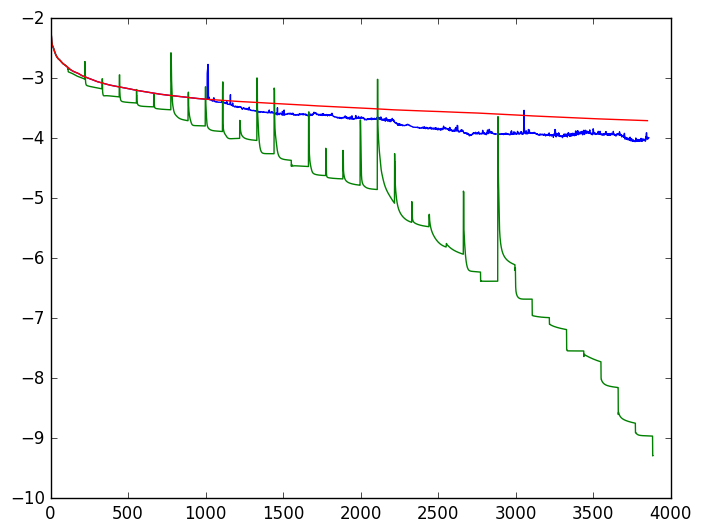Out:
1-element Array{Any,1}:
PyObject <matplotlib.lines.Line2D object at 0x32c676f90>

We see that the result of the mixed strategy between simple fixed point and AA is more efficient than AA in this particular case. Let's see the result on medium size $\sigma$ parameter.

In :
sigma = 0.01
sigmaSquared = sigma^2

coupling = GenerateCoupling(x,sigma)
cost  = GenerateCost(x,sigma)
P_init_log, P_init_simple = zeros(n,2), ones(n,2)
### Memory number for anderson acceleration:
memoryNumber = 8
### Iterations
iterations_anderson, iterations_simple, number_of_steps = 2, 100, 35
iterations_total = (iterations_simple+ iterations_anderson + memoryNumber)*number_of_steps
### Change the number of initial iterations

convergence_anderson_mixed, convergence_simple,convergence_anderson = [],[],[]
p_anderson = ParamsForFixedPointLogTrue(nu,mu,cost,sigmaSquared,convergence_anderson)
p_anderson_mixed = ParamsForFixedPointLogTrue(nu,mu,cost,sigmaSquared,convergence_anderson_mixed)
p_logtruesimple = ParamsForFixedPointLogTrue(nu,mu,cost,sigmaSquared,convergence_simple)

P,convergence_anderson = OptimizationAnderson(FixedPointLogTrue,P_init_log,iterations_burn2,iterations_total-iterations_burn2,memoryNumber,p_anderson)
P,convergence_anderson_mixed = OptimizationAndersonMixed(FixedPointLogTrue,P_init_log,iterations_simple,iterations_anderson,number_of_steps,memoryNumber,p_anderson_mixed)
P,convergence_simple = OptimizationSimpleFixedPoint(FixedPointLogTrue,P_init_log,iterations_total,p_logtruesimple)

### Plot
plot(1:length(convergence_anderson),convergence_anderson)
plot(1:length(convergence_anderson_mixed),convergence_anderson_mixed)
plot(1:length(convergence_simple),convergence_simple)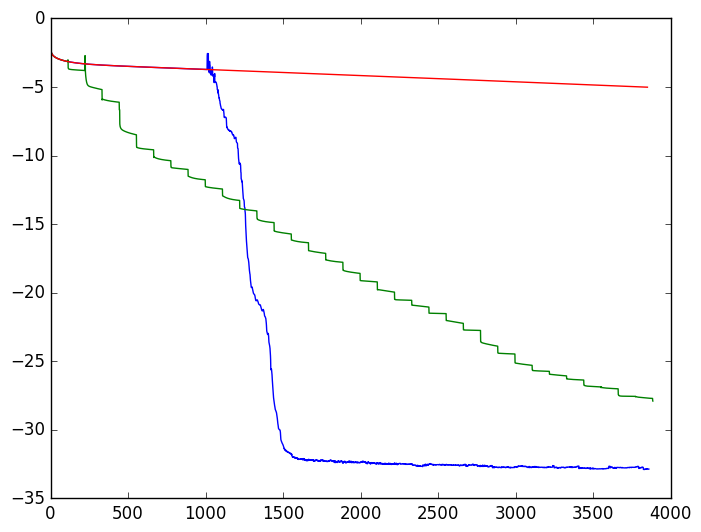Out:
1-element Array{Any,1}:
PyObject <matplotlib.lines.Line2D object at 0x32c8c1150>

As expected, this method deteriorates the convergence, but still seems to be more robust. It is maybe possible to develop a method which is adaptive in order to take advantage of the robustness of the fixed point (guaranteed in Sinkhorn) and AA.

## Multi-marginal¶

We solve Brenier generalized Euler flow using entropic regularization following Iterative Bregman Projections for Regularized Transportation Problems by Benamou et al.

They use entropic regularization on the multimarginal problem of Brenier (Generalized solutions of incompressible Euler equation) to find a kernel on the path space which has marginals at every time $t$ equal to the uniform measure and satisfies a given coupling between time $0$ and $1$.

Hereafter, we implement it on the interval and reproduce some 1D experiments by Brenier.

In :
### Simple functions for choosing the kernel ###
SetCurrentKernel(s,T) = 1 + (s==T)
### compute (pseudo) marginal (pseudo since we avoid multiplying by the Lagrange multiplier at time t
### to save computations)
function ComputePseudoMarginal(t,nT,G,P)
sequence = circshift(1:nT,-(t-1))[2:end]
temp_kernel = deepcopy(G[SetCurrentKernel(t,nT)])
for s in sequence
#println("toto",size(P[:,s]))
temp_kernel = (temp_kernel.*P[:,s]')*G[SetCurrentKernel(s,nT)]
end
return diag(temp_kernel)
end

Out:
ComputePseudoMarginal (generic function with 1 method)
In :
type ParamsForFixedPointMM <: Parameters
nu::Array{Float64,2} ### marginals
G::Array{Array{Float64,2},1} ### Kernels
convergence::Array{Float64,1}
nT::Int64
end
function BuildCouplingFinal(x,permutation,sigma)
n = length(x)
c_x = zeros(n,n)
sigma2 = sigma^2
for i=1:n
for j=1:n
c_x[i,j] = exp(-10*((x[i]-permutation[j])^2)/sigma2)
end
end
c_x
end

Out:
BuildCouplingFinal (generic function with 1 method)

Let's implement the fixed point with input/output in log-domain. Of course, in order to decrease the regularization parameter $\sigma$ a true log-domain implementation is required.

In :
### The alternate projection expressed in log domain
function FixedPointLogMM(P::Array{Float64,2},p::ParamsForFixedPointMM)
P = exp(P)
for t=1:p.nT
temp = ComputePseudoMarginal(t,p.nT,p.G,P)
if  t==Int(p.nT/2)
push!(p.convergence,log(sum(abs(temp.*P[:,Int(nT/2)] - p.nu[:,Int(nT/2)]))))
end
P[:,t] = p.nu[:,t]./temp
end
return log(P)
end

Out:
FixedPointLogMM (generic function with 1 method)
In :
function ComputeGeneralizedCoupling(t,P,G,nT)
if t==1
temp_kernel = eye(size(G))
for s in 1:nT
temp_kernel = (temp_kernel.*P[:,s]')*G[SetCurrentKernel(s,nT)]
end
return eye(size(G))/size(G)
else
temp_kernel2 = deepcopy(G[SetCurrentKernel(1,nT)])
sequence = 2:t-1
for s in sequence
temp_kernel2 = (temp_kernel2.*P[:,s]')*G[SetCurrentKernel(s,nT)]
end
temp_kernel = deepcopy(G[SetCurrentKernel(t,nT)])
sequence = t+1:nT
for s in sequence
temp_kernel = (temp_kernel.*P[:,s]')*G[SetCurrentKernel(s,nT)]
end
return (temp_kernel.*P[:,1]').*(temp_kernel2.*P[:,t]')'
end
end

Out:
ComputeGeneralizedCoupling (generic function with 1 method)

### A very simple case with large regularization parameter and coarse discretization¶

In :
using PyPlot
######## parameters #########
nT = 6  # timestep number
nX = 31# space discretization - number of points
sigma = 0.06
##sigma = 0.018 # entropic regularization parameter
x = linspace(0,1,nX) # space vector
Gx = GenerateCoupling(x, sigma) # Gaussian kernel associated with the entropic regularization
myfunc(x) = min(2*x,2*(1-x))

### definition of the target permutation.
### definition of the coupling matrix at the final time (we simply use penalization) but could be
### chosen as the permutation matrix.
GFinal = BuildCouplingFinal(x,permutation,sigma)
G = [Gx,GFinal]
P_init = zeros(nX,nT); # the Lagrange multiplier variable
nu = 1.0/nX * ones(nX,nT) # marginal constraints
convergence = []
p = ParamsForFixedPointMM(nu,G,convergence,nT)
iterations = 3000

### Simple fixed point
P,convergence_simple = OptimizationSimpleFixedPoint(FixedPointLogMM,P_init,iterations,p)

### plot
plot(1:length(convergence_simple),convergence_simple)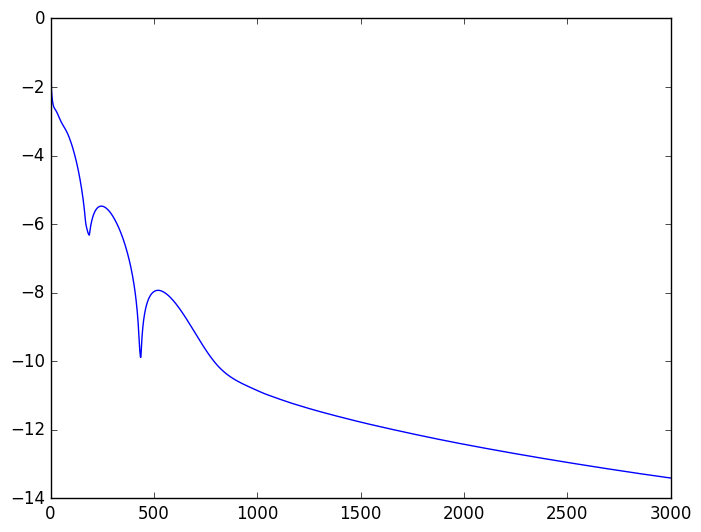WARNING: Method definition myfunc(Any) in module Main at In:9 overwritten at In:9.


Above is the result of standard alternate projection algorithm.

We now compute the coupling between time $0$ and time $t$ to illustrate the optimization result.

In :
myexp(x) = x
i = 6
coupling = ComputeGeneralizedCoupling(i,exp(P),G,nT)
println("max ",maximum(coupling))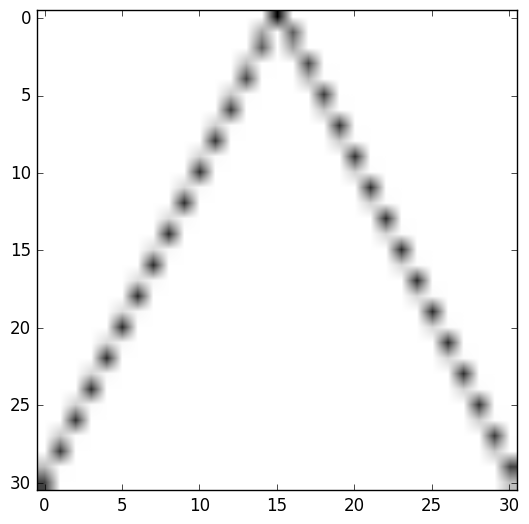max 0.032209103694063046

WARNING: Method definition myexp(Any) in module Main at In:1 overwritten at In:1.

Out:
PyObject <matplotlib.image.AxesImage object at 0x328a23410>
In :
memoryNumber = 8
iterations_burn2,iterations_anderson, iterations_simple, number_of_steps = 100,2, 100, 20
iterations_total = (iterations_simple+ iterations_anderson + memoryNumber)*number_of_steps
P_init = zeros(nX,nT);
convergence_anderson_mixed, convergence_simple,convergence_anderson = [],[],[]
p_anderson = ParamsForFixedPointMM(nu,G,convergence_anderson,nT)
p_anderson_mixed = ParamsForFixedPointMM(nu,G,convergence_anderson_mixed,nT)
p_logtruesimple = ParamsForFixedPointMM(nu,G,convergence_simple,nT)

P,convergence_anderson = OptimizationAnderson(FixedPointLogMM,P_init,iterations_burn2,iterations_total-iterations_burn2,memoryNumber,p_anderson)
P,convergence_anderson_mixed = OptimizationAndersonMixed(FixedPointLogMM,P_init,iterations_simple,iterations_anderson,number_of_steps,memoryNumber,p_anderson_mixed)
P,convergence_simple = OptimizationSimpleFixedPoint(FixedPointLogMM,P_init,iterations_total,p_logtruesimple)

### Plot
plot(1:length(convergence_anderson),convergence_anderson)
plot(1:length(convergence_anderson_mixed),convergence_anderson_mixed)
plot(1:length(convergence_simple),convergence_simple)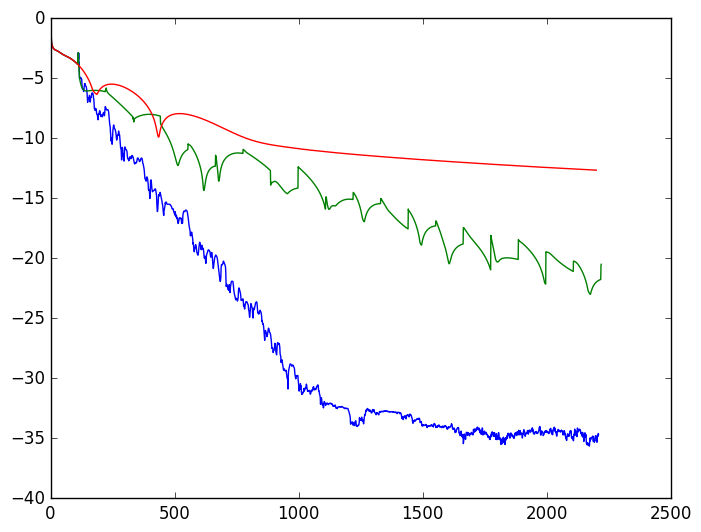Out:
1-element Array{Any,1}:
PyObject <matplotlib.lines.Line2D object at 0x328639c50>

On the experiment above, AA greatly improves the convergence and the mixed strategy is less efficient.

Let us now decrease the regularization parameter $\sigma$ and increase the discretization; the same behaviour is observed.

In :
nT = 8  # timestep number
nX = 101# space discretization - number of points
sigma = 0.025
##sigma = 0.018 # entropic regularization parameter
x = linspace(0,1,nX) # space vector
Gx = GenerateCoupling(x, sigma) # Gaussian kernel associated with the entropic regularization
myfunc(x) = min(2*x,2*(1-x))

### definition of the target permutation.
### definition of the coupling matrix at the final time (we simply use penalization) but could be
### chosen as the permutation matrix.
GFinal = BuildCouplingFinal(x,permutation,sigma)
G = [Gx,GFinal]
P_init = zeros(nX,nT); # the Lagrange multiplier variable
nu = 1.0/nX * ones(nX,nT)

memoryNumber = 8
iterations_burn2,iterations_anderson, iterations_simple, number_of_steps = 100,4, 100, 30
iterations_total = (iterations_simple+ iterations_anderson + memoryNumber)*number_of_steps
P_init = zeros(nX,nT);
convergence_anderson_mixed, convergence_simple,convergence_anderson = [],[],[]
p_anderson = ParamsForFixedPointMM(nu,G,convergence_anderson,nT)
p_anderson_mixed = ParamsForFixedPointMM(nu,G,convergence_anderson_mixed,nT)
p_logtruesimple = ParamsForFixedPointMM(nu,G,convergence_simple,nT)

P,convergence_anderson = OptimizationAnderson(FixedPointLogMM,P_init,iterations_burn2,iterations_total-iterations_burn2,memoryNumber,p_anderson)
P,convergence_anderson_mixed = OptimizationAndersonMixed(FixedPointLogMM,P_init,iterations_simple,iterations_anderson,number_of_steps,memoryNumber,p_anderson_mixed)
P,convergence_simple = OptimizationSimpleFixedPoint(FixedPointLogMM,P_init,iterations_total,p_logtruesimple)

### Plot
plot(1:length(convergence_anderson),convergence_anderson)
plot(1:length(convergence_anderson_mixed),convergence_anderson_mixed)
plot(1:length(convergence_simple),convergence_simple)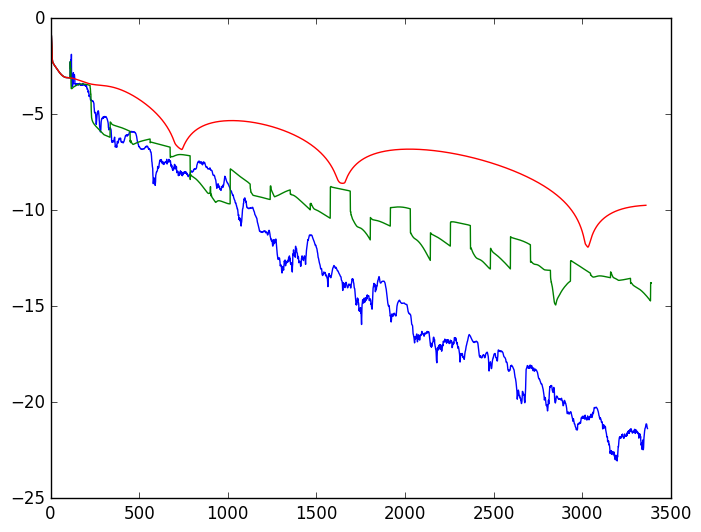WARNING: Method definition myfunc(Any) in module Main at In:7 overwritten at In:7.

Out:
1-element Array{Any,1}:
PyObject <matplotlib.lines.Line2D object at 0x3283bad50>

It appears that decreasing again $\sigma$ deteriorates again the convergence and one should increase the number of iterations accordingly.

### Conclusion¶

On these experiments, Anderson acceleration greatly improves the standard Sinkhorn iteration method. However, the results are not so impressive on Brenier's multi-marginal problem although it clearly helps the convergence. In Brenier's multi-marginal problem, a lot of computational time is needed to reach the "asymptotic linear" convergence, where AA is more efficient.

To finish, let's try to get crispier results.

In :
nT = 16 # timestep number
nX = 101# space discretization - number of points
sigma = 0.015
# entropic regularization parameter
x = linspace(0,1,nX) # space vector
Gx = GenerateCoupling(x, sigma) # Gaussian kernel associated with the entropic regularization
myfunc(x) = min(2*x,2*(1-x))

### definition of the target permutation.
### definition of the coupling matrix at the final time (we simply use penalization) but could be
### chosen as the permutation matrix.
GFinal = BuildCouplingFinal(x,permutation,sigma)
G = [Gx,GFinal]
P_init = zeros(nX,nT); # the Lagrange multiplier variable
nu = 1.0/nX * ones(nX,nT)

memoryNumber = 8
iterations_burn2,iterations_total = 1000,5000
P_init = zeros(nX,nT);
convergence_anderson = []
p_anderson = ParamsForFixedPointMM(nu,G,convergence_anderson,nT)

P_anderson,convergence_anderson = OptimizationAnderson(FixedPointLogMM,P_init,iterations_burn2,iterations_total-iterations_burn2,memoryNumber,p_anderson)

### Plot
plot(1:length(convergence_anderson),convergence_anderson)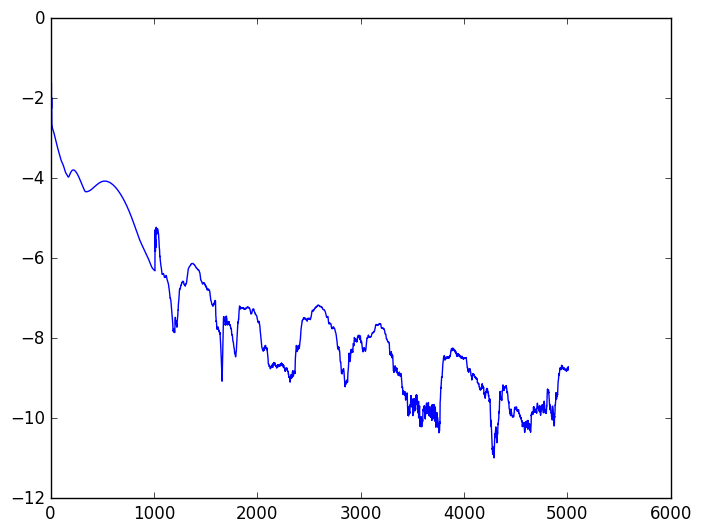WARNING: Method definition myfunc(Any) in module Main at In:7 overwritten at In:7.

Out:
1-element Array{Any,1}:
PyObject <matplotlib.lines.Line2D object at 0x32977fa50>
In :
myexp(x) = x
i = 8
coupling = ComputeGeneralizedCoupling(i,exp(P_anderson),G,nT)
println("max ",maximum(coupling))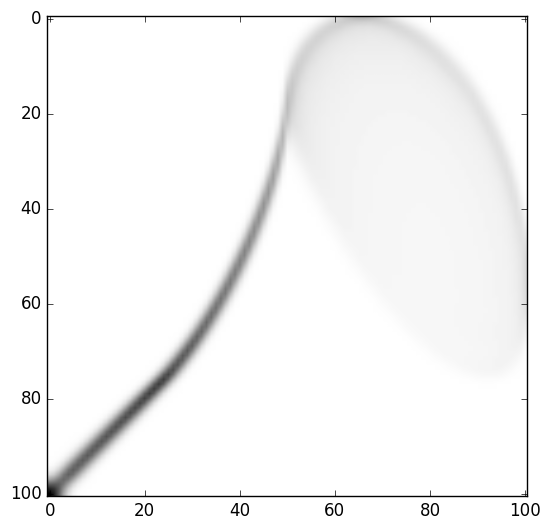max 0.003249354496016794

WARNING: Method definition myexp(Any) in module Main at In:1 overwritten at In:1.

Out:
PyObject <matplotlib.image.AxesImage object at 0x3285e0350>
In :
nT = 16 # timestep number
nX = 101# space discretization - number of points
sigma = 0.015
# entropic regularization parameter
x = linspace(0,1,nX) # space vector
Gx = GenerateCoupling(x, sigma) # Gaussian kernel associated with the entropic regularization
myfunc(x) = min(2*x,2*(1-x))

### definition of the target permutation.
### definition of the coupling matrix at the final time (we simply use penalization) but could be
### chosen as the permutation matrix.
GFinal = BuildCouplingFinal(x,permutation,sigma)
G = [Gx,GFinal]
P_init = zeros(nX,nT); # the Lagrange multiplier variable
nu = 1.0/nX * ones(nX,nT)

memoryNumber = 8
iterations_burn2,iterations_total = 2000,8000
P_init = zeros(nX,nT);
convergence_anderson = []
p_anderson = ParamsForFixedPointMM(nu,G,convergence_anderson,nT)

P_anderson,convergence_anderson = OptimizationAnderson(FixedPointLogMM,P_init,iterations_burn2,iterations_total-iterations_burn2,memoryNumber,p_anderson)

### Plot
plot(1:length(convergence_anderson),convergence_anderson)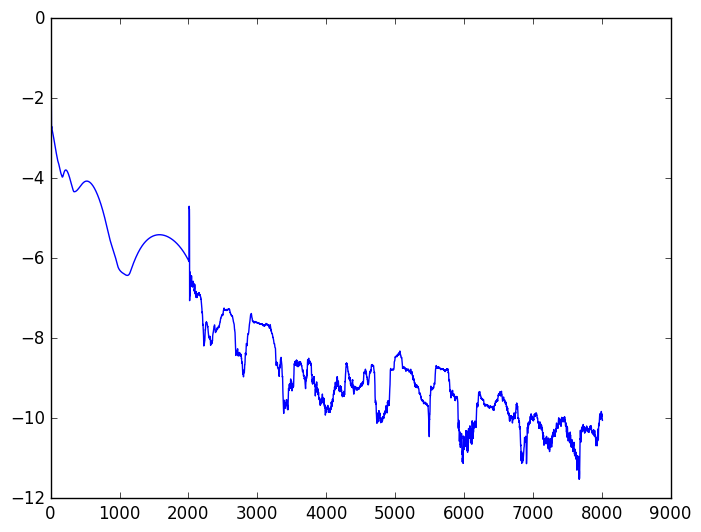Out:
1-element Array{Any,1}:
PyObject <matplotlib.lines.Line2D object at 0x3220a5210>
In [ ]: